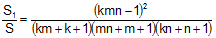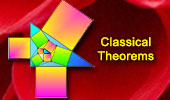Routh's Theorem 2: Triangle, Cevians, Ratio, AreasThe figure shows a triangle ABC of area S with the cevians AA', BB', and CC' so that AB'/B'C = m, BC'/AC' = n, and CA'/A'B = k. Prove that the ratio of areas between triangles DEF and ABC is.Edward Routh (1831-1907), was an English mathematician. Read more. See Also:Routh's Theorem 1. Triangle, Cevians, Ratio of Areas. Routh's Theorem 3. Triangle, Cevians, Ratio of Areas. Routh's Theorem 4. Triangle, Cevians, Ratio of Areas.
 Home | Search | Geometry | Routh's Theorem | Post a comment or solution | Email | by Antonio Gutierrez